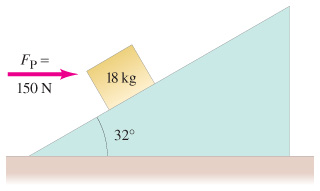# Problem: A. How much work is done by the horizontal force FP = 150 N on the 18-kg block of the figure when the force pushes the block 6.0 m up along the 32 frictionless incline?B. How much work is done by the gravitational force on the block during this displacement?C. How much work is done by the normal force?D. What is the speed of the block (assume that it is zero initially) after this d is placement? [Hint: Work-energy involves net work done.]

###### FREE Expert Solution

Work done by the force in pushing an object over a distance d is given by:

$\overline{)\begin{array}{rcl}{\mathbf{W}}& {\mathbf{=}}& \mathbf{F}\mathbf{·}\mathbf{d}\\ & {\mathbf{=}}& \mathbf{F}\mathbf{d}\mathbf{c}\mathbf{o}\mathbf{s}\mathbf{\theta }\end{array}}$

A.

In this case, the applied force, Fp = 150N

Displacement, d = 6.0 m

θ = 32°

Substituting to our work equation gives:

$\begin{array}{rcl}\mathbf{W}& \mathbf{=}& \mathbf{F}\mathbf{d}\mathbf{c}\mathbf{o}\mathbf{s}\mathbf{\theta }\\ & \mathbf{=}& \mathbf{\left(}\mathbf{150}\mathbf{\right)}\mathbf{\left(}\mathbf{6}\mathbf{.}\mathbf{0}\mathbf{\right)}\mathbf{c}\mathbf{o}\mathbf{s}\mathbf{\left(}\mathbf{32}\mathbf{°}\mathbf{\right)}\end{array}$

80% (168 ratings)###### Problem Details

A. How much work is done by the horizontal force FP = 150 N on the 18-kg block of the figurewhen the force pushes the block 6.0 m up along the 32 frictionless incline?

B. How much work is done by the gravitational force on the block during this displacement?

C. How much work is done by the normal force?

D. What is the speed of the block (assume that it is zero initially) after this d is placement? [Hint: Work-energy involves net work done.]

Frequently Asked Questions

What scientific concept do you need to know in order to solve this problem?

Our tutors have indicated that to solve this problem you will need to apply the Net Work & Kinetic Energy concept. You can view video lessons to learn Net Work & Kinetic Energy. Or if you need more Net Work & Kinetic Energy practice, you can also practice Net Work & Kinetic Energy practice problems.

What professor is this problem relevant for?

Based on our data, we think this problem is relevant for Professor Hodby's class at UCB.